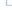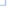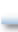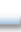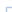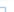# ps_circle

(PECL ps:1.1.0-1.3.5)

ps_circleDraws a circle

### Description

bool ps_circle ( resource \$psdoc , float \$x , float \$y , float \$radius )

Draws a circle with its middle point at (x , y ). The circle starts and ends at position (x +radius , y ). If this function is called outside a path it will start a new path. If it is called within a path it will add the circle as a subpath. If the last drawing operation does not end in point (x +radius , y ) then there will be a gap in the path.

### Parameters

psdoc

Resource identifier of the postscript file as returned by ps_new().

x

The x-coordinate of the circle's middle point.

y

The y-coordinate of the circle's middle point.

radius

The radius of the circle

### Return Values

Returns TRUE on success or FALSE on failure.

### See Also